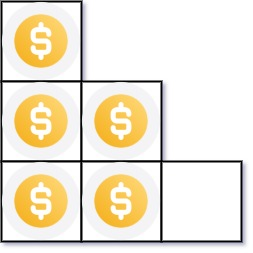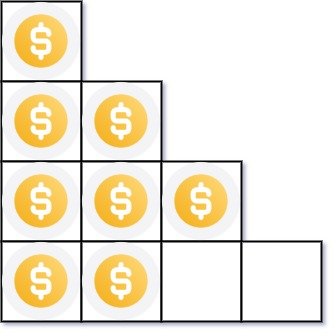# 441. Arranging Coins LeetCode

## 441. Arranging Coins

You have `n` coins and you want to build a staircase with these coins. The staircase consists of `k` rows where the `ith` row has exactly `i` coins. The last row of the staircase may be incomplete.

Given the integer `n`, return the number of complete rows of the staircase you will build.

Example 1:```Input: n = 5
Output: 2
Explanation: Because the 3rd row is incomplete, we return 2.
```

Example 2:```Input: n = 8
Output: 3
Explanation: Because the 4th row is incomplete, we return 3.
```

Constraints:

• `1 <= n <= 231 - 1`

#### The solution to the above question is

We will simply add a number to the previous sum just greater by one and then check if its smaller or equal to the given number n, and track the number of steps involved. At last we will simply return the number of steps as the answer.

class Solution {
public:
int arrangeCoins(int n) {
// t is the number to which we will add a number
long long int t=0;
// start is the number that we will add every time and increase it by one
long long int start=1;
// ans will track the number of steps
int ans=0;
while(t<=n){
t=start+t;
start++;
ans++;
}
return --ans;
}
};